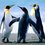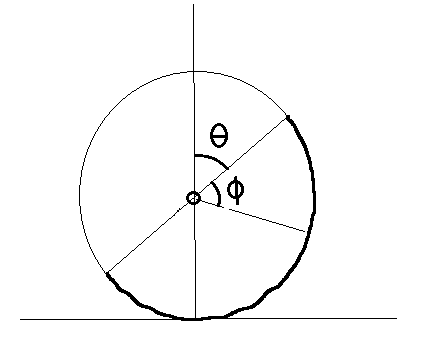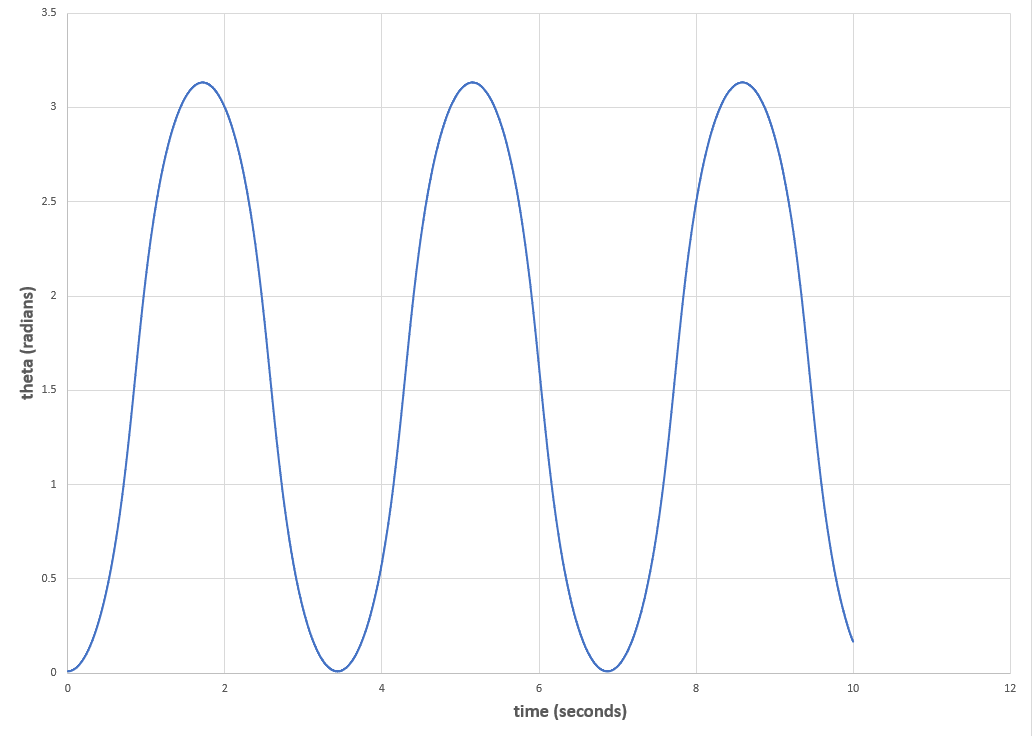# Classical mechanics problem

There is a semicircular arc, of radius $R$, mass $M$, which is placed on a ground with its diameter initially vertical, and released.The friction is sufficient to prevent slipping. Find the angular velocity and angular acceleration of the arc when it has rotated by $\theta$.Note by Kudo Shinichi
1 year, 11 months ago

This discussion board is a place to discuss our Daily Challenges and the math and science related to those challenges. Explanations are more than just a solution — they should explain the steps and thinking strategies that you used to obtain the solution. Comments should further the discussion of math and science.

When posting on Brilliant:

• Use the emojis to react to an explanation, whether you're congratulating a job well done , or just really confused .
• Ask specific questions about the challenge or the steps in somebody's explanation. Well-posed questions can add a lot to the discussion, but posting "I don't understand!" doesn't help anyone.
• Try to contribute something new to the discussion, whether it is an extension, generalization or other idea related to the challenge.

MarkdownAppears as
*italics* or _italics_ italics
**bold** or __bold__ bold
- bulleted- list
• bulleted
• list
1. numbered2. list
1. numbered
2. list
Note: you must add a full line of space before and after lists for them to show up correctly
paragraph 1paragraph 2

paragraph 1

paragraph 2

[example link](https://brilliant.org)example link
> This is a quote
This is a quote
    # I indented these lines
# 4 spaces, and now they show
# up as a code block.

print "hello world"
# I indented these lines
# 4 spaces, and now they show
# up as a code block.

print "hello world"
MathAppears as
Remember to wrap math in $$ ... $$ or $ ... $ to ensure proper formatting.
2 \times 3 $2 \times 3$
2^{34} $2^{34}$
a_{i-1} $a_{i-1}$
\frac{2}{3} $\frac{2}{3}$
\sqrt{2} $\sqrt{2}$
\sum_{i=1}^3 $\sum_{i=1}^3$
\sin \theta $\sin \theta$
\boxed{123} $\boxed{123}$

Sort by:

@Azimuddin Sheikh @Ram Mohith Here is my full solutionSuppose that the semicircle begins upright ($\theta = 0$), with the center of the diameter at $x = 0$. The semicircle then proceeds to roll without slipping.

Coordinates of center of diameter (which would be the center of the circle if the circle was full):

$x_C = R \, \theta \\ y_C = R$

Coordinates of a point on the semicircle ($\theta$ is effectively our state variable; each point has a constant $\phi$):

$x = R \, \theta + R \, sin (\theta + \phi) \\ y = R + R \, cos (\theta + \phi) \\ \dot{x} = R \,\dot{\theta} + R \, \dot{\theta} \, cos (\theta + \phi) \\ \dot{y} = -R \, \dot{\theta} \, sin (\theta + \phi)$

Velocity of a point on the semicircle:

$v^2 = \dot{x}^2 + \dot{y}^2 = 2 \, R^2 \, \dot{\theta}^2 + 2 \, R^2 \, \dot{\theta}^2 cos (\theta + \phi)$

Infinitesimal mass:

$dm = M \frac{d \phi}{\pi} = \frac{M}{\pi} \, d \phi$

Infinitesimal kinetic energy:

$dE = \frac{1}{2} \, dm \, v^2 = \frac{1}{2} \, \frac{M}{\pi} \, d \phi \, \Big [2 \, R^2 \, \dot{\theta}^2 + 2 \, R^2 \, \dot{\theta}^2 cos (\theta + \phi) \Big] \\ = \frac{M \, R^2 \, \dot{\theta}^2}{\pi} [1 + cos(\theta + \phi) ] \, d \phi$

Total kinetic energy:

$E =\frac{M \, R^2 \, \dot{\theta}^2}{\pi} \int_0^{\pi} [1 + cos(\theta + \phi) ] \, d \phi \\ = \frac{M \, R^2 \, \dot{\theta}^2}{\pi} [\pi -2 \, sin \theta ]$

Infinitesimal gravitational potential energy:

$dU = dm \, g \, y = \frac{M g}{\pi} \, d \phi \, [R + R \, cos (\theta + \phi)] = \frac{M g R}{\pi} \, [1 + cos (\theta + \phi)] \, d \phi$

The gravitational potential energy integral is the same as the kinetic energy integral, except for the constant:

$U = \frac{M g R}{\pi} [\pi -2 \, sin \theta ]$

System Lagrangian:

$L = E - U = \frac{M \, R^2 \, \dot{\theta}^2}{\pi} [\pi -2 \, sin \theta ] - \frac{M g R}{\pi} [\pi -2 \, sin \theta ]$

Equation of motion:

$\frac{d}{dt} \frac{\partial{L}}{\partial{\dot{\theta}}} = \frac{\partial{L}}{\partial{\theta}}$

Evaluation step one:

$\frac{\partial{L}}{\partial{\dot{\theta}}} = \frac{ 2 M \, R^2 \, \dot{\theta}}{\pi} [\pi -2 \, sin \theta ]$

Evaluation step two:

$\frac{d}{dt} \frac{\partial{L}}{\partial{\dot{\theta}}} = \frac{ 2 M \, R^2 }{\pi} \Big [\dot{\theta} (-2 \, cos \theta \, \dot{\theta} ) + \ddot{\theta} (\pi -2 \, sin \theta ) \Big ] \\ = -\frac{ 4 M \, R^2 }{\pi} \, cos \theta \, \dot{\theta}^2 + 2 M \, R^2 \, \ddot{\theta} -\frac{ 4 M \, R^2 }{\pi} \, sin \theta \, \ddot{\theta}$

Evaluation step three:

$\frac{\partial{L}}{\partial{\theta}} = \frac{M \, R^2 \, \dot{\theta}^2}{\pi} [-2 \, cos \theta ] - \frac{M g R}{\pi} [ -2 \, cos \theta ] \\ = -\frac{2 M \, R^2 \, \dot{\theta}^2}{\pi} cos \theta + 2 \frac{M g R}{\pi} cos \theta$

Equating:

$-\frac{ 4 M \, R^2 }{\pi} \, cos \theta \, \dot{\theta}^2 + 2 M \, R^2 \, \ddot{\theta} -\frac{ 4 M \, R^2 }{\pi} \, sin \theta \, \ddot{\theta} = -\frac{2 M \, R^2 \, \dot{\theta}^2}{\pi} cos \theta + 2 \frac{M g R}{\pi} cos \theta \\ 2 M \, R^2 \, \ddot{\theta} -\frac{ 4 M \, R^2 }{\pi} \, sin \theta \, \ddot{\theta} = \frac{2 M \, R^2 \, \dot{\theta}^2}{\pi} cos \theta + 2 \frac{M g R}{\pi} cos \theta \\ R \pi \, \ddot{\theta} - 2 R \, sin \theta \, \ddot{\theta} = R \dot{\theta}^2 cos \theta + g cos \theta$

Final result:

$\ddot{\theta} = \frac{R \dot{\theta}^2 cos \theta + g \, cos \theta}{R \pi - 2 R \, sin \theta }$

Here is a plot of theta vs time (generated from numerical integration) for a semicircle diameter of 2 meters (the height of a tall man). The period is about 3.5 seconds, which seems reasonable for an object of that size. You can see that it rocks back and forth between $\theta = 0$ (standing on one end of the diameter) and $\theta = \pi$ (standing on the other end).- 1 year, 11 months ago

Outstanding sir got it now , thx very much. Pls see the new discussion note I started of collisions. Pls see

- 1 year, 11 months ago

If it's alright with you, I'll post a problem based on this one (the semicircle)

- 1 year, 11 months ago

Yeah sure sir post it .

- 1 year, 10 months ago

Is this question same as that one in Mechanics

- 1 year, 11 months ago

Yeah was same

- 1 year, 11 months ago

I got the value of angular velocity as, $\omega = 2 \sqrt{\dfrac{g(1 - \cos \theta)}{3R}}$ but I don't know whether it is correct or not. Here is the way I did it.

As the friction is sufficient to prevent slipping the semicircular disk can be assumed to be performing pure rotation about the bottom most point let's say "O". Now, to find $\omega$ I used the concept of the law of conservation of energy. The energy changes that take place here are only rotational kinetic energy and change in potential energy. To find the potential energy first we have to calculate by how much height did the center of mass displaced. By fixing the initial position of center of mass as datum line after rotating through $\theta$ the center of mass will move down by $h$ and I found it to be $h = R(\cos \theta - 1)$ which will be negative since the center of mass moved below the datum line. After rotating through $\theta$ the disk will acquire an angular velocity $\omega$ so it will have a rotational kinetic energy of $\dfrac12 I_o \omega^2$ where moment of inertia of the semicircular disc about point "O" is $I_o = \dfrac{mR^2}{2} + mR^2 = \dfrac32 mR^2$ by parrallel axis theorem. By law of conservation of energy, \begin{aligned} \text{Total final energy} & = \text{Total initial energy} \\ \dfrac12 I_o \omega^2 + mgh & = 0 \\ \dfrac12 I_o \omega^2 + mgR(\cos \theta - 1) & = 0 \\ \dfrac12 I_o \omega^2 = mgR(1 - \cos \theta) & \\ \dfrac34 mR^2 \omega^2 = mgR(1 - \cos \theta) & \\ \omega^2 = \dfrac{4g(1 - \cos \theta)}{3R} & \\ \therefore \boxed{\omega = 2\sqrt{\dfrac{g(1 - \cos \theta)}{3R}}} & \\ \end{aligned}

I think this may be wrong so please inform me if it is.

- 1 year, 11 months ago

Did you account for the fact that the center of mass is not on the diameter?

- 1 year, 11 months ago

Ok. I forgot about that. But is the procedure correct.

- 1 year, 11 months ago

That will make the math more complicated, particularly because the distance used in the parallel axis calculation will vary with the angular position. And you'll have to calculate the COM moment as well, which won't be trivial. But I would be interested in comparing your revised result with my result.

- 1 year, 11 months ago

Ok. Thank you. But I am thinking of very small values of theta so that we can make necessary assumptions and arrive at the correct result.

- 1 year, 11 months ago

(@Steven Chase) sir can u check this out

- 1 year, 11 months ago

This looks like an interesting one. I'll take a look at it

- 1 year, 11 months ago

Here is the result after the first derivation. $\theta$ is the angle we care about, and $\phi$ is another angle that I used to do the integrals. I will derive it again tomorrow, and if I get the same thing, I will post the full solution in a separate note. The bottom line seems to be that the differential equation requires numerical integration to solve. Even a small angle approximation doesn't make things any better.$\ddot{\theta} = \frac{R \, cos \theta \, \dot{\theta}^2 + g \, cos \theta}{R \pi - 2 R \, sin \theta}$

- 1 year, 11 months ago

Also, I have another doubt. Will the answer vary whether we use a full arc or semicircular arc.

- 1 year, 11 months ago

It has to be a half arc. In my drawing, the thick line represents the actual mass, and the thin line is just there for reference.

- 1 year, 11 months ago

@Steven Chase sir , can we do it from bottommost part , taking torque of mg and equating it to I alpha .( between note that bottommost point is non inertial frame) ?? Also what will be the angular velocity if done by my method ) ??

- 1 year, 11 months ago

Ram.mohith u have solved problem in non inertial frame r u sure ?? Of your answer

- 1 year, 11 months ago

Also pls see this problem too : @Steven Chase sir @Ram Mohith https://goiit.com/t/doubt-from-mechanics/39565/15?u=azimuddin_sheikh in this link

- 1 year, 11 months ago

I am unable to see the problem as a dialogue box asking to login is blocking the question. Can you write the question here or keep a screenshot.

- 1 year, 11 months ago

How to post a screenshot here ?? Between r u sure of your answer bro as u solved in non inertial frame

- 1 year, 11 months ago

You can start to create a dummy problem and put a picture in there. Then copy the text for the picture, abort the problem creation, and put the picture text in this note. By the way, for the first problem you asked about, do you know what the answer is supposed to be?

- 1 year, 11 months ago

No. My answer is wrong. I just had a try. The two main flaws in my solution are the position of center of mass which I took is wrong and the distance I took to apply parallel axis theorem is wrong.

- 1 year, 11 months ago

@Steven Chase sir , can we do it from bottommost part , taking torque of mg and equating it to I alpha .( between note that bottommost point is non inertial frame) ?? Also what will be the angular velocity if done by my method ) ?? Between from bottommost point what we have to do when we r now solving in non inertial frame ?? Is my approach correct?? @Ram Mohith bro leaving those two flaws r u sure its correct as such bottommost point is non inertial frame?? Check this link for solution to this problem : http://artofproblemsolving.com/community/c164h1785345p11808151 ( what he wrote in A5, is it correct,is it so easy )?? Steven chase sir and ram Mohith bro

- 1 year, 11 months ago

As I told Ram earlier, I would be interested in comparing (numerically) his result to my result, after he revises his. Yours sounds similar to his, except using torque instead of energy. I will eventually get around to posting my full solution, but I did it the way I did in order to make as few assumptions as possible. The solution on the website isn't fleshed out very much.

- 1 year, 11 months ago

Also, in my drawing, is that the "theta" that you want? The angle between the vertical and the edge of the semicircle?

- 1 year, 11 months ago

Yes sir that's the only angle we have to consider. Between in the solution posted in that link , isn't it so easy just use energy equation to get angular velocity sir ,is it incorrect ??or something more is need to be considered? Is there any assumption made in solution in that link ??

- 1 year, 11 months ago

That seems like it may work, but the trick is that the moment of inertia (about the point touching the ground) varies with the angle. Also, the center of mass does not lie on the diameter.

- 1 year, 11 months ago